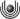Aktuelle Publikationen

• D. Choi, S. Lim, T. Mühlenbruch, W. Raji: Series expansion of the period function and representations of Hecke operators (pdf, 499804) [externer Link]

Abstract: The period polynomial of a cusp form of an integral weight plays an important role in the number theory. In this paper, we study the period function of a cusp form of real weight. We obtain a series expansion of the period function of a cusp form of real weight for SL(2;Z) by using the binomial expansion. Furthermore, we study two kinds of Hecke operators acting on cusp forms and period functions, respectively. With these Hecke operators we show that there is a Hecke-equivariant isomorphism between the space of cusp forms and the space of period functions. As an application, we obtain a formula for a certain L-value of a Hecke eigenform by using the series expansion of its period function.

(Erscheint in Jorunal of Number Theory; externe Links: doi:10.1016/j.jnt.2016.07.020, preprint http://staff.aub.edu.lb/~wr07/Papers/CMRL.pdf)

• J. Kerner and T. Mühlenbruch: Two interacting particles on the half-line [externer Link]

Abstract: In the case of general compact quantum graphs, many-particle models with singular two-particle interactions were introduced by Bolte and Kerner [J. Phys. A: Math. Theor. 46, 045206 (2013); 46, 045207 (2013)] in order to provide a paradigm for further studies on many-particle quantum chaos. In this note, we discuss various aspects of such singular interactions in a two-particle system restricted to the half-line ℝ+. Among others, we give a description of the spectrum of the two-particle Hamiltonian and obtain upper bounds on the number of eigenstates below the essential spectrum. We also specify conditions under which there is exactly one such eigenstate. As a final result, it is shown that the ground state is unique and decays exponentially as sqrt{x^2+y^2} → ∞.FernUniversität in Hagen, Lehrgebiet Stochastik, 58084 Hagen, Tel.: +49 2331 987-2283